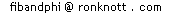#### Aims

This is a page about generalising the Fibonacci Formula F(n)=F(n-1)+F(n-2) to a similar relationship but on the powers of the Fibonacci numbers: F(n)2 and F(n)3 and so on.

#### Background

The material on this page assumes that you already know a little about the Fibonacci Numbers from Fibonacci Numbers, Golden Section and the Golden String (which contains a much fuller treatment of Fibonacci numbers and their mathematical properties) and are familiar with polynomials and algebra at school level age 14-15.
This page is an Appendix to those web pages, covering extra material aimed at the level of students in school aged 15-17.

## Introduction

On this page we will introduce you to the Fibonacci Factorial function F!(n) and the Fibonomial array of numbers, so called because the Fibonomials are like the Binomial coefficients of Pascal's Triangle.
From the formula F(n)=F(n-1) + F(n-2) connectng three consecutive Fibonacci numbers, we find one connecting four consecutive F(n)2 terms, five consecutive F(n)3 terms, six consecutive F(n)4 terms and so on. These are called Recurrence Relations (or Recursion Equations) since they are not explicit formulae that give F(n)2 or F(n)3 in terms of n but in terms of some preceding terms in the F(n)p series. Finally we uncover the formula for connecting any p+2 consecutive p-th powers of Fibonacci numbers. You will also discover the powerful mathematical technique of examining a series by making the numbers in the series into the coefficients of a polynomial in x, called the Power Series of the sequence.
Often we can then find a simple mathematical expression for that polyomial called its Generating Function. This is similar to relating the (infinite) sequence 3,7,0,3,7,0,... with first the (infinite) decimal fraction 0.370370370... and then the (finite) fraction 10/27.

# Recurrence Relations

For the Fibonacci numbers themselves, if we know any two consecutive terms then we can add them to get the next which we express in mathematical notation as:
F(n) = F(n-1) + F(n-2)
We have also seen that there are many such series, the General Fibonacci series, and we need to state two starting terms to determine one particular sequence. For the Fibonacci numbers 0, 1, 1, 2, 3, 5, 8, 13, ... , these two starting conditions could be:-
F(0)=0, F(1)=1
For the Lucas Numbers: 2, 1, 3, 4, 7, 11, ..., we have
L(n) = L(n-1) + L(n-2); L(0)=2, L(1)=1
Such a formula for a series which defines each number in it in terms of some combination of previous terms is called a recurrence relation or recurrence formula.
For a series S this means defining S(n) in terms of some combination of previous terms S(n-1), S(n-2) down to S(0), though it may not always use all of the previous terms to define the next.

On this page we find simple recurrence relations for the F(n)2 series, F(n)3 series and the other powers of the Fibonacci numbers. The pattern we end up with turns out to have some nice parallels with the Binomial Coefficients (the numbers in Pascal's Triangle) and introduces us to the Fibonomials.

# Powers of Fibonacci Numbers

In this section we look at the series of squares of Fibonacci numbers then later at the cubes and higher powers.

## Squares of Fibonacci Numbers and a Recurrence Relation

For the squares of the Fibonacci numbers we have:
 n F(n) F(n)2 1 2 3 4 5 6 7 ... 1 1 2 3 5 8 13 ... 1 1 4 9 25 64 169 ...
The recurrence relationship for F(n)2 is not too easy to spot. However, it has been found already:
F(n)2 = 2 F(n – 1)2 + 2 F(n – 2)2 – F(n – 3)2
The recurrence relation tells us how to find F(n)2 using the previous 3 terms of the F(n)-squared series: F(n-1)2, F(n-2)2, F(n-3)2
• double the latest term: 2 F(n-1)2
• double the one before the latest: 2 F(n-2)2 and add these
• finally subtract the term two before the latest: F(n-3)2
For example: to find the next term after 1, 1, 4, 9 :
• double the latest term: 2 F(n-1)2 = 2×9 = 18
• double the one before the latest: 2 F(n-2)2 = 2×4 = 8 and add these
• finally subtract the term two before the latest: F(n-3)2 = 1
25 = 2×9 + 2×4 - 1 = 25 which is correct since it is F(5)2
Repeat the process now to find the next term following 1, 1, 4, 9, 25:
64 = 2x25 + 2x9 - 4
Now we have 1, 1, 4, 9, 25: the next is
2x64 + 2x25 - 9 = 128 + 50 - 9 = 169 which is indeed F(7)2=132
It is easy to use a spreadsheet to generate the F(n)-squared series using the previous 3 cells in the list and so generate the series of squares of Fibonacci numbers.

It will be useful later to look at this equation with all the Fibonacci terms on one side:

F(n)2 – 2 F(n – 1)2 – 2 F(n – 2)2 + F(n – 3)2 = 0
Let's have a look at the Cubes of the Fibonacci numbers next.

## The Cubes of the Fibonacci Numbers and their Recurrence Relation

 n F(n) F(n)3 1 2 3 4 5 6 7 ... 1 1 2 3 5 8 13 ... 1 1 8 27 125 512 2197 ...
The rule here was found by Zeitlin and Parker and published in the Fibonacci Quarterly in 1963:
F(n)3 = 3 F(n – 1)3 + 6 F(n – 2)3 – 3 F(n – 3)3 – F(n – 4)3
Check it for yourself or using a spreadsheet again.

Again, we write it with all the terms on one side as we did for the Squares:

F(n)3 – 3 F(n – 1)3 – 6 F(n – 2)3 + 3 F(n – 3)3 + F(n – 4)3 = 0
Our list of recurrence relationships for the powers from 1 to 3 now looks like this:
F(n) – F(n – 1) – F(n – 2) = 0
F(n)2 – 2 F(n – 1)2 – 2 F(n – 2)2 + F(n – 3)2 = 0
F(n)3 – 3 F(n – 1)3 – 6 F(n – 2)3 + 3 F(n – 3)3 + F(n – 4)3 = 0
Does the pattern generalise?

## Fourth Powers

Does the pattern generalise? If so the next one would look something like
F(n)4 + A F(n – 1)4 + B F(n – 2)4 + C F(n – 3)4 + D F(n – 4)4 + E F(n – 5)4 = 0
Like the earlier formulae, we want this to hold for all values of n.
We have 5 numbers A, B, C, D and E to find and we can find them by solving some simultaneous equations if we put in some small values for n. Since our recurrence will hold for all values of n, it must also hold for the simple cases of n=5:
F(5)4 + A F(4)4 + B F(3)4 + C F(2)4 + D F(1)4 + E F(0)4 = 0 Using numeric Fib values:
54 + A 34 + B 24 + C 14 + D 14 + E 04 = 0 Evaluating the powers:
625 + A 81 + B 16 + C 1 + D 1 + E 0 = 0
625 + 81A + 16B + C + D = 0
We can do this for n=6, 7, 8 and 9 also so that we have 5 equations in the 5 variables A, B, C, D and E and we can then solve these.
It's a bit of a task doing this by hand and a computer algebra package (such as Derive, Maple or Mathematica) saves us a lot of time and, let's face it, is probably more accurate than doing it by hand too!
Either way we get the following values for the coefficients:
A = –5, B = –15, C = 15, D = 5, E = –1
If we put these 5 numbers into the suggested recurrence relationship above, we can evaluate a few more terms and see that it holds not just for n = 5, 6, 7, 8 and 9 but it looks as if it holds for all values of n:
F(n)4 – 5 F(n – 1)4 – 15 F(n – 2)4 + 15 F(n – 3)4 + 5 F(n – 4)4 – F(n – 5)4 = 0
We will prove this later.

## Fifth Powers

The same method gives a recurrence formula for F(n)5:
F(n)5 – 8 F(n – 1)5 – 40 F(n – 2)5 + 60 F(n – 3)5 + 40 F(n – 4)5 – 8 F(n – 5)5 – F(n – 6)5 = 0

# The Recurrence Relations for Powers of Fibonaccis

In this section we show how to find a general formula for the recurrence relations for F(n)p using p for the power. Our list now looks like this:
F(n) – F(n – 1) – F(n – 2) = 0
F(n)2 – 2 F(n – 1)2 – 2 F(n – 2)2 + F(n – 3)2 = 0
F(n)3 – 3 F(n – 1)3 – 6 F(n – 2)3 + 3 F(n – 3)3 + F(n – 4)3 = 0
F(n)4 – 5 F(n – 1)4 – 15 F(n – 2)4 + 15 F(n – 3)4 + 5 F(n – 4)4 – F(n – 5)4 = 0
F(n)5 – 8 F(n – 1)5 – 40 F(n – 2)5 + 60 F(n – 3)5 + 40 F(n – 4)5 – 8 F(n – 5)5 – F(n – 6)5 = 0
If we look at the coefficients in these recurrences we have:
 1 -1 -1 1 -2 -2 1 1 -3 -6 3 1 1 -5 -15 15 5 -1 1 -8 -40 60 40 -8 -1
These coefficients are called the Fibonomial numbers because they share many properties with the binomial numbers (binomial coefficients). So let's look at the Binomial numbers in more detail.

## The Binomial Coefficients

Perhaps this reminds you of Pascal's Triangle which is usually shown in one of these two forms:
col= 0 1 2 3 4 5 1 1 1  1 1 1 1  2  1 1 2 1 1  3  3  1 1 3 3 1 1  4  6  4  1 1 4 6 4 1 1  5  10  10  5  1 1 5 10 10 5 1 ... ...
On an earlier page at this site we looked at the patterns in this table and found the Fibonacci numbers.
The rows of Pascal's Triangle are expansions of powers of a binomial term - a term with two parts such as (1+x):-
 (1+x)0 = 1 (1+x)1 = 1 +x (1+x)2 = 1 + 2 x + 1 x2 (1+x)3 = 1 + 3 x + 3 x2 + 1 x3 (1+x)4 = 1 + 4 x + 6 x2 + 4 x3 + 1 x4 (1+x)5 = ...
Here the x's are numbers (any number) rather than terms in a series that our recurrence relations deal with. The formula for the pattern in our new table is similar to that of the Binomial numbers.
In Pascal's triangle, the binomial coefficient on row n and column k is written as follows and has the following formula:
 ( n ) k
=
 n! = n . (n–1) . (n–2) ... (n–k+1)k! (n–k)! k. (k–1). ... 2. 1
n! is the factorial of n (n is a whole number) and is just the product of all the numbers from n down to 1. For example,
1! = 1
2! = 2 × 1 = 2
3! = 3 × 2 × 1 = 6
4! = 4 × 3 × 2 × 1 = 24
5! = 5 × 4 × 3 × 2 × 1 = 120
You might think that since this definition involves a fraction then for some values of n and k then the result will be a fraction not a whole number – but it is always a whole number! The numbers on the bottom will always "cancel" or be factors of those on the top.
For instance,
 ( 5 ) 3
=
 5! = 5 × 4 × 3 × 2 × 1 = 5 × 4 = 103! (5 – 3)! (3 × 2 × 1) (2 × 1) 2 × 1
and the number on the row labelled 5 and column labelled 3 in Pascal's Triangle is 10.

Note that we have a special case when n is 0 or n=k: we have to let 0! mean 1 in the binomial coefficient formula to get the numbers shown in Pascal's Triangle.

Sometimes, to save room on a printed page, the binomial coefficient is written as nCk or nCk or even as binomial(n,k).
It is read as "n choose k" because given n different objects it is the number of ways we can choose k of them.

For instance, given the 5 objects A, B, C, D and E, we can choose a set of three of them in the following 5C3 = 10 ways:
ABC ABD ABE ACD ACE ADE BCD BCE BDE CDE

The pattern in our table of coefficients for recurrence relations of F(n)P needs two more ideas first: the Fibonacci Factorial and the Fibonomial.

## The Fibonacci Factorial

Instead of n! which is the product of all the numbers from n down to 1, we now introduce the Fibonacci Factorial n !F which is the product of the Fibonacci numbers from F(n), F(n-1) down to F(1).
There is no commonly accepted notation for the Fibonacci Factorial. Simon Plouffe uses a nicer notation: FF(n) although even better is F!(n). Since the latter is more readable, we will use F!(n) on this page.
n !F or F!(n) stands for F(n) F(n-1) F(n-2) ... F(2) F(1)
For example:
F!(1) = 1
F!(2) = F(2) F(1) = 1 × 1 = 1
F!(3) = F(3) F(2) F(1) = 2 × 1 × 1 = 2
F!(4) = F(4) F(3) F(2) F(1) = 3 × 2 × 1 × 1 = 6
F!(5) = F(5) F(4) F(3) F(2) F(1) = 5 × 3 × 2 × 1 × 1 = 30
Here is a table of the first 20 Fibonacci Factorials with their factors: Note: It makes formulae simpler if we let F!(0) be a special case and define F!(0) to be 1 instead of the more logical value of 0.
nF!(n)F!(n) factors
01 1
1 1 1
2 1 1
3 2 2
4 6 2 3
5 30 2 3 5
6 240 24 3 5
7 3120 24 3 5 13
8 65520 24 32 5 7 13
9 2227680 25 32 5 7 13 17
10 122522400 25 32 52 7 11 13 17
11 10904493600 25 32 52 7 11 13 17 89
12 1570247078400 29 34 52 7 11 13 17 89
13 365867569267200 29 34 52 7 11 13 17 89 233
14 137932073613734400 29 34 52 7 11 132 17 29 89 233
15 84138564904377984000 210 34 53 7 11 132 17 29 61 89 233
16 83044763560621070208000 210 35 53 72 11 132 17 29 47 61 89 233
17 132622487406311849122176000 210 35 53 72 11 132 17 29 47 61 89 233 1597
18 342696507457909818131702784000 213 35 53 72 11 132 172 19 29 47 61 89 233 1597
19 1432814097681520949608649339904000 213 35 53 72 11 132 172 19 29 37 47 61 89 113 233 1597
20 9692987370815489224102512784450560000 213 36 54 72 112 132 172 19 29 37 41 47 61 89 113 233 1597
This is A003266 in Sloane's Online Encyclopedia of Integer Sequences.

## A Formula for the Fibonomial

Now we define a new form of "binomial coefficient" but using this definition of factorial instead. We will use a subscript "F" after the binomial brackets to distinguish it from the binomial coefficient itself:
 ( n )F k
=
 F!(n)F!(k) F!(n – k)
=
 F(n)F(n–1)...F(n–k+1)F(n–k)F(n–k–1)...F(2)F(1)F(k)F(k–1)...F(2)F(1)   F(n–k)F(n–k–1)...F(2)F(1)
 There is no universal notation for the Fibonomial. D Knuth and others use double brackets: (( n )) k
A simple alternative is to write fibonomial(n,k) which has the advantage of using less space on the page. Here is a table of some values of the fibonomial
k
0 1 2 3 4 5 6 7
n1 1 1
2 1 1 1
3 1 2 2 1
4 1 3 6 3 1
5 1 5 15 15 5 1
6 1 8 40 60 40 8 1
7 1 13 104 260 260 104 13 1
The above appears as A010048 in Sloane's Online Encyclopedia of Integer Sequences (OEIS).

To get the signed Fibonomials, all the numbers in every other pair of columns after the first column are negated:

k
0 1 2 3 4 5 6 7
n1 1 –1
2 1 –1 –1
3 1 –2 –2 1
4 1 –3 –6 3 1
5 1 –5 –15 15 5 –1
6 1 –8 –40 60 40 –8 –1
7 1 –13 –104 260 260 –104 –13 1
The signed table is A055870 in Sloane's OEIS.

## How can we tell if a Fibonomial is negative in this table?

The negative columns occur in pairs between two positive columns. How can we incorporate this into our formulae? We can use the rounding functions and here we introduce you two three of them: round, floor, ceiling.

### The Rounding Functions: round, floor and ceiling

The most common rounding funciton is perhaps round(x) which "rounds" a real-number value x to the nearest whole number.
There are two other kinds of rounding too: round-up or ceiling(x) and round-down or floor(x).

For example, when we pay for an item in a shop, we must round-up the price to present a whole number of pound coins:
an item costing £3.80 can be paid for with 4 pound coins. Even an item costing £3.01 must be rounded-up to 4 pound coins.
We write this as ceiling(3.80) = ceiling(3.01) = 4

On the other hand, having bought an item and paid for it, giving change involves the round-down function:
If the customer is needs £3.80 in change, we give 3 pound coins (and 80 pence).
Similarly for change to the value of £3.01.
We write this as floor(3.80) = floor(3.01) = 3

The third everyday case is when a friend, admiring your wise purchase, asks how much it cost. We would often just give a rough figure to the nearest whole number of pounds).

• If it cost £3.80 we would say "around 4 pounds"
• if £3.01 then "about 3 pounds".
We have rounded to the nearest whole number of pounds.
We write this as round(3.80)=4; round(3.01)=3 If the cost was £3.50 then we would have to decide if we said £3 or £4 since both 3 and 4 are equally close to 3.50.

### Rounding negative values

When we round a negative value, we have to be careful about the floor and ceiling values. We adopt the common convention that:
floor(x) is the next whole number less than (more negative than) x.
ceiling(x) is the next whole number greater than (more positive than) x.
round(x) is still the closest whole number and we must decide what to do about values such as 3.5 and -7.5 which are exactly half-way between two whole number values.
For whole numbers x, the result of all of these functions is x itself.
e.g.
Result -> 210-1-2
floorfloor(2.9)
floor(2.1)
floor(2)
floor(1.9)
floor(1.3)
floor(1.1)
floor(1)
floor(0.8)
floor(0.4)
floor(0)
floor(-0.2)
floor(-0.8)
floor(-1)
floor(-1.2)
floor(-1.9)
floor(-2)
ceilingceiling(1.9)
ceiling(1.3)
ceiling(1.1)
ceiling(2)
ceiling(0.8)
ceiling(0.4)
ceiling(1)
ceiling(-0.2)
ceiling(-0.9)
ceiling(0)
ceiling(-1.2)
ceiling(-1.9)
ceiling(-1)
ceiling(-2.8)
ceiling(-2.4)
ceiling(-2)
roundround(2.1)
round(1.9)
round(2)
round(1.3)
round(0.8)
round(1)
round(0.4)
round(-0.2)
round(0)
round(-0.8)
round(-1.2)
round(-1)
round(-1.9)
round(-2.3)
round(-2)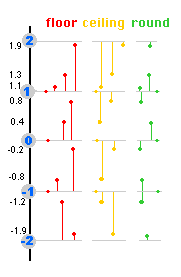### Why call these functions floor and ceiling?

Because we can image an object held at height n on a vertical number line with "floors" at the whole number levels.
For the floor function, the object is heavy so it falls to the next whole number floor level if it is not already at a whole number on the line.
For the ceiling function, imagine a helium-filled balloon which rises from height n to the next whole number above it if not already at a whole number height.
Tis is shown in the diagram at the side of the Table above.

### Floor or Trunc?

For positive values of x floor(x) means the same as "forget anything after the decimal point" and may be called trunc for "truncate" on some calculators. Beware of using the "trunc" button on negative values as trunc(-2.8) may produce -2 by truncating the value at the decimal point whereas floor(-2.8) is -3.

### A formula for the sign of a Fibonomial

We want a pair of values to be positive as k increases, then a pair of negative and so on across each row.
The columns with the negative signs are k=1,2, (not 3 or 4) 5,6, (not 7 or 8) 9, 10, .... we can do this using powers of -1 and the pairing of values is done using a rounding function.

Here is what happens is we use k/2 in the floor function as our power of (-1):

 k k/2 floor(k/2) (-1)floor(k/2) 0 1 2 3 4 5 6 7 8 0 0.5 1 1.5 2 2.5 3 3.5 4 0 0 1 1 2 2 3 3 4 1 1 -1 -1 1 1 -1 -1 1
This is not quite what we want: we can
• EITHER use k+1 instead of k: (-1)floor((k+1)/2)
• OR use the ceiling function: (-1)ceiling(k/2)
 k k/2 ceiling(k/2) (-1)ceiling(k/2) 0 1 2 3 4 5 6 7 8 0 0.5 1 1.5 2 2.5 3 3.5 4 0 1 1 2 2 3 3 4 4 1 -1 -1 1 1 -1 -1 1 1

This now gives us a formula (a way of predicting) the sign of the coefficient in the recurrence relations. Now we look at the coefficients themselves, the Fibonomials.

## A Recurrence Relation for the Fibonomials

Each binomial coefficient can be found by using the two numbers above it when each row is centred or, when the table is laid out with vertical columns, the number above and the number to the left of that one.
Those two numbers and merely added:
col= 0 1 2 3 4 5 1 1 1  1 1 1 1  2  1 1 2 1 1  3  3  1 1 3 3 1 1  4  6  4  1 1 4 6 4 1 1  5  10  10  5  1 1 5 10 10 5 1 ... ...
There is a way of calculating each Fibonomial in terms of the two on the row above it too, but it involves the Fibonacci numbers too:
 Row 4 Row 5 1 3 6 3 1 |1| \  5  \ |0| \  3  \ |1| \  2  \ |1| \  1  \ |2| \ 1  \ 1x1=1 1x5+3x0=5 3x3+6x1=15 6x2+3x1=15 3x1+1x2=5 1x1=1
To find the Fibonomials on row 5 from those on row 4, the two series of numbers used are 1 0 1 1 2 ... and ... 5 3 2 1 1
The second series (using the number above) starts with F(n) on row n and goes down.
Row n=5:
 k=0: 0 + 1×F(-1) = 1; k=1: 1×F(5) + 3×F(0) = 5; k=2: 3×F(4) + 6×F(1) = 15; k=3: 6×F(3) + 3×F(2) = 15; k=4: 3×F(2) + 1×F(3) = 5; k=5: 1×F(1) + 0 = 1
D E Knuth (The Art of Computer Programming, Vol. 1 Fundamental Algorithms, Section 1.2.8 Exercise 27) gives the formula for this method as
fibonomial( n, k ) = F( n – k + 1 ) fibonomial( n – 1, k – 1 ) + F( k – 1 ) fibonomial( n – 1, k )
Note that the indexes of the two Fibonacci multipliers always sum to n, the row number of the (new) Fibonomial.
Compare this with the much simpler formula for the binomial coefficients where we merely add the two numbers on the row above to get each term:
 ( n ) k
=
 ( n – 1 ) k
+
 ( n – 1 ) k – 1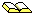A Sequence of Power Formulas Bro A Brousseau The Fibonacci Quarterly vol 6 (1968) pages 81-83
took essentially the same approach as shown above and found a recursive method of generating the power formula, but did not explicitly give the recursion.
Later on this page we will find Fibonomial formula that are very similar to their binomial counterparts, but first, let's put all the above together and show the complete formula for our Fibonacci Power recurrence relations.

# A Formula for the Fibonacci Powers' Recurrence Relations

D E Knuth (ibid. Exercise 30) also gives the full formula for the recurrences we found for the Fibonacci Powers, and our form here is slightly simpler than his but is essentially the same form:
 p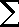k=0
 ( p )F k
(-1)ceiling(k/2)F(n – k)p-1
= 0, if p>0

# More on the Fibonomials and the Binomials

## Symmetric Rows

The rows of Pascal's Triangle and the rows in our Fibonomial Triangle are symmetrical.
In mathematical terms we express this as:
binomial( n, k ) = binomial( n, n – k)
Fibonomial( n, k ) = Fibonomial( n, n – k)

## Relationship with term before

Each term on a row can be computed directly from the term before it on the same row:
 ( n ) k
=
 n – k + 1k
 ( n ) k – 1
, k0
 ( n )F k
=
 F( n – k + 1)F( k )
 ( n )F k – 1
, k0

## Relationship with term above

Each term can be computed from the one "above" (in the same column):
 ( n ) k
=
 nn – k
 ( n – 1 ) k
, kn
 ( n )F k
=
 F( n )F( n – k )
 ( n – 1 )F k
, kn

# Generating Functions for Powers of Fibonacci Numbers

Sometimes it helps to solve a mathematical problem if we relate a series to a polynomial in x with the series as the coefficients. Such a series is called a Power Series and often we can find a simple expression for the (infinite) power series. Here are some examples:

## A Generating Function for the Fibonacci Numbers

The Fibonacci numbers (omitting 0): 1, 1, 2, 3, 5, ...
As a Power Series: 1 + 1 x + 2 x2 + 3 x3 + 5 x4 + ...
But
 11 – x – x2
=  1 + 1 x + 2 x2 + 3 x3 + 5 x4 + ...
so the generating function for the Fibonacci numbers is
 11 – x – x2
On an earlier page we gave a simple proof and saw how we could use this series and its shorter generating function to find decial fractions which are the Fibonacci numbers .
By letting x= 0.1 in the generating function we have a fraction 1/ (1 - 0.1- 0.01 = 100/(100-10-1) = 100/89.
Looking at this fraction in terms of its power series, we have 0.1 + 0.01 + 0.002 + 0.0003+ ... = 0.11235...
Letting x=0.01 and x=0.001 give the decimal fractions 0.101020305081321... and 0.1001002003005008013021... and so on.

What happened to the missing first term of 0? If you want to restore it, then the power series is 0 + 1 x + 1 x2 + 2 x3 + 3 x4 + ...
All we have done is multiply the series by x!
So, with the extra 0 term at the start, the generating function is
 x1 – x – x2

## A Generating Function for the Squares of the Fibonacci Numbers

Can we find a generating function for the series F(n)2? Yes - here it is:
The series F(n)2: 1, 1, 22 = 4, 32 = 9, 52 = 25, ...

As a Power Series: 1 + 1 x + 4 x2 + 9 x3 + 25 x4 + ...
The generating function is
 1 – x1 – 2 x – 2 x2 + x3

## More Generating Functions for Powers of Fibonacci Numbers

Here is a table summarising the above results and extending them
Fibonacci termsGenerating Function
x0x1x2x3x4x5 ...
F(n)112358...
 11 – x – x2
F(n)2 1 1 4 9 25 64 ...
 1 – x1 – 2 x – 2 x2 + x3
F(n)3 1 1 8 27 125 512 ...
 1 – 2 x – x21 – 3 x – 6 x2 + 3 x3 + x4
F(n)4 1 1 16 81 625 4096 ...
 1 – 4 x – 4 x2 + x31 – 5 x – 15 x2 + 15 x3 + 5 x4 – x5
F(n)5 1 1 32 243 3125 32768 ...
 1 – 7 x – 16 x2 + 7 x3 + x41 – 8 x – 40 x2 + 60 x3 + 40 x4 – 8 x5 – x6
Did you notice that the polynomials in the denominators all have coefficients which are just a row of the Fibonomial Table?

# More Generating Functions and the Fibonomials

If we return to our Fibonomial Table, they have some more surprises for us when we see what polynomials they form when we use them as coefficients, that is, what are the generating functions for the Fibonomial rows and columns.

## Fibonomial Row Generating Functions

With the signs, we have the following table, witht he k value telling us which coefficient of x we use for the numbers in its column in order to make a polynomial in x - its Generating Function (G.F.)
nFibonomial Row as a GFFactors
11–1x1–x
21–1x–1x21–x–x2
31–2x–2x2+1x31+x1–3x+x2
41–3x–6x2+3x3+1x41+x–x21–4x–x2
51–5x–15x2+15x3+5x4–1x51–x1+3x+x21–7x+x2
61–8x–40x2+60x3+40x4–8x5–1x61–x–x21+4x–x21–11x–x2
71–3x–104x2+260x3+260x4–104x5–13x6+1x71+x1–3x+x21+7x+x21–18x+x2
The factors of the GF polynomials have some interesting relationships:
• All the factors are linear or quadratic
• The linear factors are (1 ± x)
• Each new row introduces one new quadratic factor
• All the factors of the GF of row n-2 are, with some sign changes, factors of the GF of row n
• The quadratic factors are all of the form 1 ± A x ± x2
• The coefficients of x in the quadratic factors are the Lucas Numbers:  0 1 2 3 4 5 6 ... 2 1 3 4 7 11 18 ...
The factors that are "copied" from the row two before have changed the sign of the coefficients of x, but the constant terms (1) and the x2 terms remain unaltered.
An easy way to express this is that the "x" has been replaced by "-x".
If FGF(n,x) means the Fibonomial Generating Function (i.e. the polynomial) of row n in the Table above, then we can express all the observations in the list as follows:
FGF(1, x) = 1 – x
FGF(2, x) = 1 – x – x2
FGF(n, x) = FGF(n–2, –x) ( 1 – L(n – 1)x + (–1)n+1 x2), n > 2
This result is given as a comment on A055870. noting that the result is derivable from the Knuth and Riordan references which are at the foot of this page.

### Row Factors and Phi

The quadratic factors can be factored further into two real roots as follows where, as usual on these pages Phi = (√5 + 1)/2, phi = (√5 – 1)/2
 –1 – x + x2 = (x – Phi)(x – (–phi)) –1 + x + x2 = (x + Phi)(x + (–phi)) 1 – 3 x + x2 = (x – Phi2)(x – (–phi)2) 1 + 3 x + x2 = (x + Phi2)(x + (–phi)2) –1 – 4 x + x2 = (x – Phi3)(x – (–phi)3) –1 + 4 x + x2 = (x + Phi3)(x + (–phi)3) 1 – 7 x + x2 = (x – Phi4)(x – (–phi)4) 1 + 7 x + x2 = (x + Phi4)(x + (–phi)4) ... (–1)n – L(n) + x2 = (x – Phin)(x – (–phi)n) (–1)n + L(n) x + x2 = (x + Phin)(x + (–phi)n)
Underlying this is this simple formula for the Lucas Numbers L(n) in terms of the golden section numbers Phi and phi:
L(n) = Phin + (–phi)n

## Fibonomial Column Generating Functions

We will compare the generating functions for the columns of Pascal's Triangle (binomial coefficients) with the generating functions for the Fibonomials.

### Generating Functions for Binomial Columns

The columns of Pascal's Triangle are the generating functions of some simple polynomials:
Pascal's
Triangle
c o l
012345...
r
o
w
01
111
2121
31331
414641
515101051
........................
GF
 11–x
 1(1–x)2
 1(1–x)3
 1(1–x)4
 1(1–x)5
 1(1–x)6
...
Here, 1/(1–x) = 1 + x + x2 + x3 + x4 +... so the coefficients are 1, 1, 1, 1, 1, ... which is the first column.
1/(1–x)2 = 1 + 2 x + 3 x3 + 4 x4 + 5 x5 +... and the coefficients here are 1, 2, 3, 4, 5, .... which is the second column, and so on.

Note that if we let x have a numerical value, then they are only true if –1 < x < 1. However, we are merely using the expansion as a convenient mathematical expression which is a "holder" for the infinite series of numbers.
For instance, let x=0.1 = 1/10 in 1/(1–x). This fraction is then 1/0.9=10/9=1 + 1/9. The GF expansion gives 1 + 1×0.1 + 1×0.01 + ... = 1.11111... which is correct as the decimal fraction for 10/9.
Try it for other values to see a numerical fraction and its expansion.
If we let x=0.01=1/100 in the second column's GF, we have the decimal fraction 1.02030405060708091011... and the GF fraction is 1/(1–0.01)2=(100/99)2=10000/9801

### Generating Functions for Fibonomial Columns

Here is a table of the columns of Fibonomials as coefficients of (rational) functions of x:
Fibonomialsc o l
012345...
r
o
w
01
111
2111
31221
413631
515151551
61840604081
........................
GF
 11 – x
 11–x–x2
 11–2x–2x2+x3
 11–3x–6x2+3x3+x4
 11–5x–15x2+15x3+5x4–x5
... ...
Did you notice that the polynomials in the denominators have coefficients which are themselves Fibonomials?
Stuart Eugene Thiel proved it but then found an earlier proof was published in The generalized Fibonomial matrix by E. Kilic in European Journal of Combinatorics, vol 31 (2010) pages 193-209.

####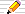Things to do1. Find a recurrence relationship between every other Fibonacci number squared:
F(1)2, F(3)2, F(5)2, ,...
2. Find a recurrence relationship between the other series of alternate Fibonacci squares:
F(2)2, F(4)2, F(6)2, ,...
3. What about every third Fibonacci cubed? There are three series to try:
F(0)3, F(3)3, F(6)3, ,...
F(1)3, F(4)3, F(7)3, ,...
F(2)3, F(5)3, F(8)3, ,...
Is the recurrence the same in each case?
5. What is the general pattern?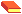The Art of Computer Programming D E Knuth, Volume 1: Fundamental Algorithms (now in its Third Edition, 1997).The product of sequences with a common linear recursion formula of order 2 Dov Jarden, Theodor Motzkin, Riveon Lematematika vol 3 (1949), pages 25-27 and 38 (Hebrew with English summary); and in English translation :Fibonacci Powers and a Fascinating Triangle Dale K Hathaway, Stephen L. Brown The College Mathematics Journal 28 (1997), pages 124-128.Recurring Sequences Dov Jarden, (second edition 1966), pages 30-33 - now out of print - and in the first edition 1958, pages 42-45.Generating functions for powers of Fibonacci numbers by J. Riordan, Duke. Math. J. vol 29 (1962) pages 5-12.© 1996-2021 Dr Ron Knott